Looking for revision notes that are specific to the exam board you are studying? If so, click the links below to view our condensed, easy-to-understand revision notes for each exam board, practice exam question booklets, mindmap visual aids, interactive quizzes, PowerPoint presentations and a library of past papers directly from the exam boards.

# Electrons

## Key Information & Summary

• Electrons are subatomic particles found in the shells around the nucleus in an atom
• There are four sub-shells. Sub-shell s has one orbital, p has three orbitals, d has five orbitals, and f has seven orbitals.
• The number of protons and electrons are equal in an atom but different in cation and anions.
• Ionisation energy is the energy change measured when one electron is removed from a gaseous atom.
• An electron has a larger angle of deflection than a proton.
• Electrons play a very important role in bonding.
• Electron affinity is the addition of one electron into a gaseous atom

## Definition of electron

Electrons a sub-atomic particles. They have a charge of negative one elementary charge  and a mass that is 1/1836 that of a proton. It is a very small piece of matter and energy. It was discovered in 1897 by J.J. Thompson.  They are known as elementary particles because they can no be broken down into smaller particles. They take part in many chemical reactions and chemical bonding.

## Location of electrons

Unlike protons and neutrons which are found inside the nucleus, electrons are found outside the nucleus in the shells. Shells are also known as principle quantum numbers. Different principle quantum numbers have different number of electrons in them. The first principle quantum number has maximum two electrons. The second shell can have up to eight electrons. The third shell can accommodate maximum eighteen electrons. The fourth principle quantum number can have maximum thirty two electrons. A shell cannot accommodate more than thirty two electrons. Shell number five, six and seven will also have thirty two electrons.## Sub-shells and orbitals

Sub-shells arise due to difference in energy of electrons being in the same principle quantum numbers. There are four sub-shells (s, p, d, f). Orbital is the 3-D space in which the probability of finding electrons is maximum.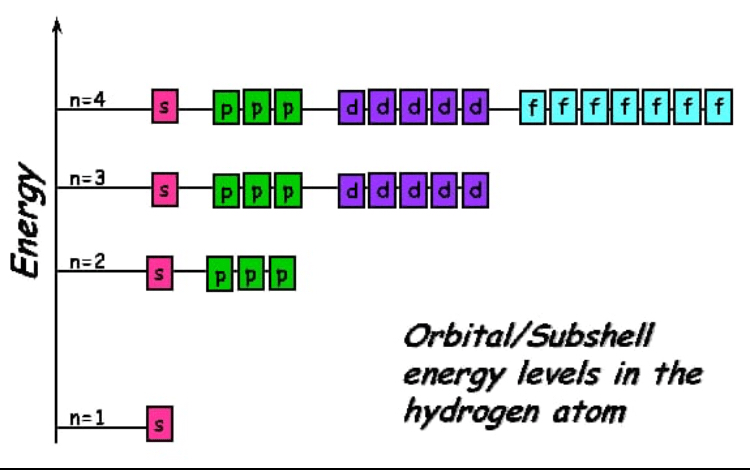An orbital is represented by a box 🔲. Maximum two electrons can be placed in an orbital. The two electrons in the orbital are shown by opposite arrows. This shows that in an orbital one electron has a clockwise spin, the other has an anticlockwise spin to minimise repulsion. Sub-shell s has one orbital, p has three orbitals, d has five orbitals, f has seven orbitals.

In this diagram n shows the shell number. Electrons would be placed in lower energy orbitals first and then in the higher energy orbitals in ascending energy order.

Shielding effect:  The inner shell electrons shields the nucleus and thus attraction for valence electron is decreased. Shielding is same across a period as the number of inner shell electrons is same. Down the group shielding effect increases due to the increase in the number of electrons in the inner shells.

## Electrons in an atom, anion and cation

In an atom the number of electrons is equal to the number of protons. This is why an atom has no charge and is neutral. In an anion the number of electrons are more than the number of protons. This is why anions have negative charge. In cations, the number of protons are more than the number of electrons. This is why cations have a positive charge.## Ionisation energy

First ionisation energy: It is the minimum energy required to remove one mol of electrons from one mol gaseous atoms to form one mol gaseous uni-positive cation.

Na(g) —> Na+1(g) + 1e-

Second ionisation energy: It is the minimum energy required to remove one mol of electrons from one mol of gaseous uni-positive cation to form one mol of gaseous dispositive  cation.

Na+1(g)  —>  Na+2(g) + 1e-

Ionisation energies increase across a period and decrease down the group. Reactivity of metals is dependant on the ionisation energies of metals. Since the ionisation energies decrease down the group, the reactivity of group one and group two metals increase down the group.

## Deflection of subatomic particlesNeutrons will not deflect as they have no charge. Electrons will show the most deflection as they have a very small mass. Electrons will have the largest angle of deflection. There will be less deflection of protons due to greater mass. Protons will have a small angle of deflection.

## Chemical bonding and electron

Ionic bond: It is the bond formed by the complete transfer of electrons from a metal to a non-metal. Nature of force is the electrostatic force of attraction between positive and negative ions. Ionic compounds exist as ionic lattice, not as molecules. They have no intermolecular forces of attraction between them. In solid state ions are not mobile so they do not conduct electricity. Ionic compounds conduct electricity only in molten or aqueous  state as ions are mobile. The following is the dot and cross diagram of an ionic compound Sodium chloride (NaCl).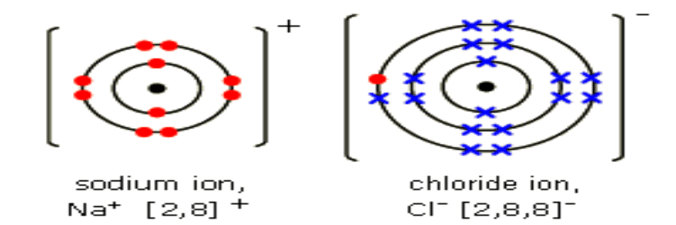Covalent bond: It is the bond formed by the mutual sharing of electron/electrons between similar or dissimilar non-metals. Nature of force is the electrostatic force of attraction between nuclei and shared electron pair. The following are dot and cross diagrams of some covalent compounds.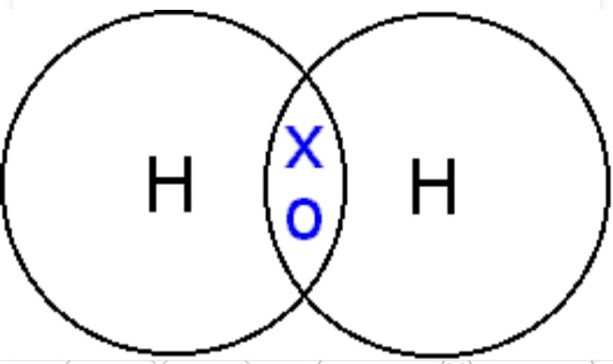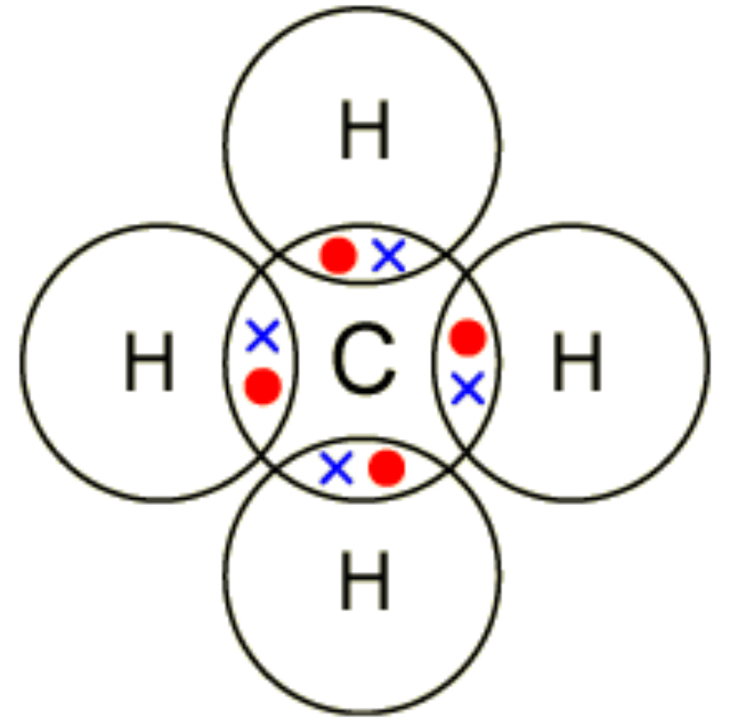Dative covalent bond: It is the bond formed by one sided sharing of lone electron pair. For dative bond formation, one atom must have a lone electron pair and the other atom must be electron deficient (need two electrons). Dative covalent bond is also known as coordinate covalent bond.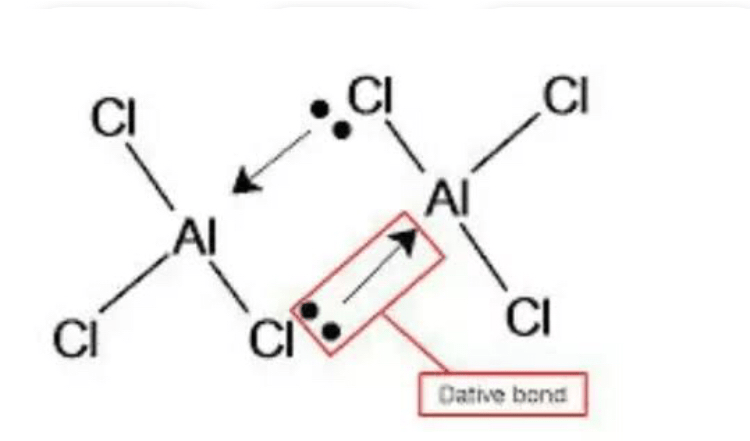Metallic bond: The electrostatic force of attraction between positive ions of metal and delocalised electrons.

Polarisation: The distortion of an electron cloud of an anion caused by a cation.

Electronegativity: The ability of an atom to attract shared electron pair from a covalent bond.

Bond polarity: When shared electron pair is unequally attracted between covalently bonded atom. Shared electron pair is more attracted by more electronegative atom, hence this atom gets partial negative charge. The less electronegative atom gets partial positive charge. Bond polarity is developed when electronegativity difference is significant (greater that 0.4).

Hydrogen bond: It is a bond formed between the lone pair of fluorine, oxygen or nitrogen of one molecule and partial positive hydrogen of another molecule which should be covalently bonded to fluorine, oxygen or nitrogen. A solute will dissolve in a solvent of they have hydrogen bonding between them.

## Electron affinity

First electron affinity: The enthalpy change measured when one mol of electrons is added to one mol of gaseous atom to form one mol of gaseous uni-negative anion. Only first electron affinity is exothermic as negatively charged electron is attracted by a neutral atom, rest of all are endothermic, as negatively charged electron is forced into anions.

O(g) + 1e- —> O-(g)

Second electron affinity: The enthalpy change measured when one mol of electron is added to one mol gaseous uni-negative anion to make one mol gaseous dl-negative anion.

O-(g) + 1e- —> O-2(g)